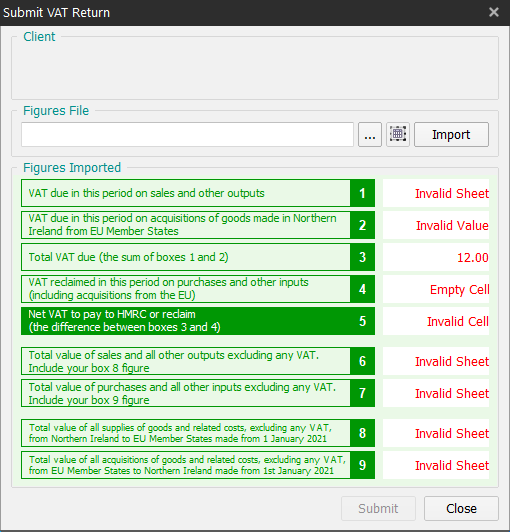# Why am I seeing errors in red after trying to import my VAT figures?

Prior to submitting a VAT return to HMRC, it is important the figures being sent are correct and what HMRC expects. For this reason Easy MTD VAT performs a number of checks on the figures it tries to import and if a check fails it is reported in the associated box in the Submit VAT Return window.Below is a list of the errors, what they mean and how to correct them:

Error Description Solution
Empty Cell The cell in the spreadsheet does not contain a value. In the Spreadsheet Settings window, specify a cell where the corresponding box figure exists in the spreadsheet.
Invalid Cell The cell format is invalid or does not exist in the spreadsheet. In the Spreadsheet Settings window, specify a cell where the corresponding box figure exists in the spreadsheet.
Invalid Sheet The sheet does not exist in the spreadsheet. In the Spreadsheet Settings window, specify the sheet name where the corresponding box figure appears in the spreadsheet. Click here for further details about sheets.
Invalid Value The cell does not contain a number or the box figure is not equal to a value that is expected based on other box figures (e.g. box 3 must be the sum of boxes 1 and 2). In the Spreadsheet Settings window, specify a cell where the corresponding box figure exists in the spreadsheet and/or ensure the box figure is calculated correctly in the spreadsheet.
Number The box figure is not equal to a value that is expected based on other box figures (e.g. box 3 must be the sum of boxes 1 and 2). Ensure the box figure is calculated correctly in the spreadsheet.

NOTE: Box figures displayed in orange will not prevent the VAT return from being submitted (unlike errors displayed in red). They are displayed in orange to indicate the box figure has been calculated by Easy MTD VAT or not used.